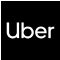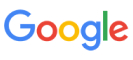New update is available. Click here to update.
Topics

# Word Search - lll

Moderate0/80
Average time to solve is 20m+1 more companies

## Problem statement

You are given character matrix of dimension N * M. Then you are given 'Q' queries, each query consists of a word 'W', and you have to tell whether it is possible to form word 'W' by choosing some adjacent characters from this matrix.

Note:

``````1. Two characters are said to be adjacent if they share a side or corner i.e. for a cell inside the matrix have 8 adjacent cells (if they exist).
2. One character in the matrix will not be considered twice for forming a word.
3. All the characters in the matrix and words consist only of uppercase English alphabets only.
``````
Detailed explanation ( Input/output format, Notes, Images )
Constraints:
``````1 <= N * M <= 12
1 <= Q <= 100
1 <= |W| <= 20
``````
Sample Input 1:
``````3 3 3
COD
RER
CODER
DONUTS
``````
Sample Output 1:
``````1
1
0
``````
Explanation of sample input 1:
``````In the matrix “CODER” can be found at [(1, 1), (1, 2), (1, 3), (2, 3), (3, 3)] and “RADE” can be found at [(3, 1), (2, 1), (2, 2), (2, 3)]. But there is no way to find “DONUTS” in the matrix.
``````
Sample Input 2:
``````2 2 2
ZO
OM
ZOO
MOOD
``````
Sample Output 2:
``````1
0
``````
Explanation of sample input 2:
``````In the matrix “ZOO” can be found at [(1, 1), (1, 2), (2, 1)]. But “MOOD” can not be found in the matrix.
``````Console